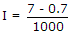Electronics and Communication Engineering - Electronic Devices and Circuits

36.

Secondary emission is always decremental.

 A. True B. False

Explanation:

Sometimes it can be useful also.

37.

In a degenerate n type semiconductor material, the Fermi level,

 A. is in valence band B. is in conduction band C. is at the centre in between valence and conduction bands D. is very near valence band

Explanation:

This is due to high level of doping.

38.

The types of carriers in a semiconductor are

 A. 1 B. 2 C. 3 D. 4

Explanation:

Holes and electrons.

39.

A potential of 7 V is applied to a silicon diode. A resistance of 1 K ohm is also in series with the diode. The current is

 A. 7 mA B. 6.3 mA C. 0.7 mA D. 0

Explanation:40.

Assertion (A): The reverse saturation current in a semiconductor diode is 4nA at 20°C and 32 nA at 50°C.

Reason (R): The reverse saturation current in a semiconductor diode doubles for every 10°C rise in temperature.

 A. Both A and R are true and R is correct explanation of A B. Both A and R are true but R is not a correct explanation of A C. A is true but R is false D. A is false but R is true